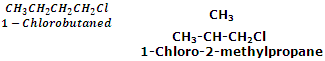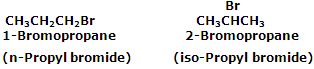How to establish nomenclature for halides?

In the common chemistry terminologies, aliphatic halogen derivatives are named as alkyl halides. The words, n-, sec-, tert-, iso-, neo-, and amyl are usually used in written in the common names. In IUPAC system, they are considered as derivatives of corresponding alkanes and are named as haloalkanes. It may be noted that the common name of any alkyl halide is written as two separate words whereas the IUPAC name of the alkyl halide is written as one word.

The dihalogen derivatives having same type of halogen atoms on the same carbon are known as germinal dihalides and are assigned common name alkylidene halides or alkylidene dihalides.

The dihalogen derivatives having the two similar halogen atoms on adjacent carbon atoms are known as vicinal dihalides and are assigned common name alkylene or alkylene dihalides.

Trihalomethnanes are called haloforms in trivial system.

Fully halogenated hydrocarbons are known as perhalohydrocarbons. For example, C2Cl6 is known as percholoroethane.

Haloarenes are named by prefixing the halogen and its position, if necessary, to the name of the parent aromatic compound.

In writing the common names, the relative positions of the substituents at 1, 2-; 1, 3- and 1, 4- positions are indicated by prefixes ortho (o-), meta (m-) and para (p-), respectively.

Isomerism in haloalkanes

Haloalkanes can exhibit the following kinds of isomerism:

Chain isomerism

The haloalkanes with four or more carbon atoms exhibit this kind of isomerism. For example,Position isomerism

The haloalkanes with three or more carbons show this kind of isomerism.

For example C3H7Br has two position isomers.#### Related Questions in Chemistry

• ##### Q :Question relatede to calculate molarity

Select the right answer of the question. What is molarity of a solution of HCl that contains 49% by weight of solute and whose specific gravity is 1.41 : (a) 15.25 (b) 16.75 (c) 18.92 (d) 20.08

• ##### Q :Haloalkanes define primary secondary

define primary secondary and tertiary alkyl halides with examples

• ##### Q :Relative lowering in vapour pressure of

Give me answer of this question. "Relative lowering in vapour pressure of solution containing non-volatile solute is directly proportional to mole fraction of solute". Above statement is: (a) Henry law (b) Dulong and Petit law (c) Raoult's law (d) Le-Chatelier's pri

• ##### Q :What is cannizaro reaction? Explain

Aldehydes which do not have  -hydrogen atom, such as formaldehyte and benzaldehyte, when heated with concentrated (50%)alkali solutio

• ##### Q :Calculation of concentration of the

Choose the right answer from following. 200ml of a solution contains 5.85 dissolved sodium chloride. The concentration of the solution will be(Na= 23: cl = 35.5 ) (a) 1 molar (b) 2 molar (c) 0.5 molar (d) 0.25 molar

• ##### Q :Degree of dissociation The degree of

The degree of dissociation of Ca(No3)2 in a dilute aqueous solution containing 14g of the salt per 200g of water 100oc is 70 percent. If the vapor pressure of water at 100oc is 760 cm. Calculate the vapor pr

• ##### Q :What are different mechanisms for

Nucleophilic substitution reactions in halides containing  - X bond may take place through either of the two different mechanisms,S<

• ##### Q :Liquid Vapour Free Energies The free

The free energy of a component of a liquid solution is equal to its free energy in the equilibrium vapour.Partial molal free energies let us deal with the free energy of the components of a solution. We use these free energies, or simpler concentration ter

• ##### Q :Production of alcoholic drinks give all

give all physical aspects in the production of alcohol

• ##### Q :Molarity 20mol of hcl solution requires

20mol of hcl solution requires 19.85ml of 0.01 M NAOH solution for complete neutralisation. the molarity of hcl solution